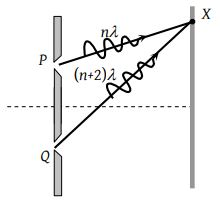Two coherent light sources S1 and S2 (λ= 6000 Å) are 1mm apart from each other. The screen is placed at a distance of 25 cm from the sources. The width of the fringes on the screen should be

(1) 0.015 cm

(2) 0.025 cm

(3) 0.010 cm

(4) 0.030 cm

Concept Questions :-

Diffraction
High Yielding Test Series + Question Bank - NEET 2020

Difficulty Level:

The figure shows a double slit experiment P and Q are the slits. The path lengths PX and QX are nλ and (n + 2) λ respectively, where n is a whole number and λ is the wavelength. Taking the central fringe as zero, what is formed at X(1) First bright

(2) First dark

(3) Second bright

(4) Second dark

Concept Questions :-

Young Double slit experiment
High Yielding Test Series + Question Bank - NEET 2020

Difficulty Level:

The Young's experiment is performed with the lights of blue (λ = 4360 Å) and green colour (λ = 5460 Å), If the distance of the 4th fringe from the centre is x, then

(1) x (Blue) = x (Green)

(2) x (Blue)> x (Green)

(3) x (Blue) < x (Green)

(4) $\frac{x\left(Blue\right)}{x\left(Green\right)}=\frac{5460}{4360}$

Concept Questions :-

Young Double slit experiment
High Yielding Test Series + Question Bank - NEET 2020

Difficulty Level:

In Young's double slit experiment, if L is the distance between the slits and the screen upon which interference pattern is observed, x is the average distance between the adjacent fringes and d being the slit separation. The wavelength of light is given by

(1) $\frac{xd}{L}$

(2) $\frac{xL}{d}$

(3) $\frac{Ld}{x}$

(4) $\frac{1}{Ldx}$

Concept Questions :-

Young Double slit experiment
High Yielding Test Series + Question Bank - NEET 2020

Difficulty Level:

In Young's experiment, light of wavelength 4000 Å is used to produce bright fringes of width 0.6 mm, at a distance of 2 meters. If the whole apparatus is dipped in a liquid of refractive index 1.5, then fringe width will be

(1) 0.2 mm

(2) 0.3 mm

(3) 0.4 mm

(4) 1.2 mm

Concept Questions :-

Young Double slit experiment
High Yielding Test Series + Question Bank - NEET 2020

Difficulty Level:

In Young's double-slit experiment, the phase difference between the light waves reaching the third bright fringe from the central fringe will be (λ =6000 Å )

(1) Zero

(2) 2$\pi$

(3) 4$\pi$

(4) 6$\pi$

Concept Questions :-

Young Double slit experiment
High Yielding Test Series + Question Bank - NEET 2020

Difficulty Level:

In two separate set - ups of the Young's double slit experiment, fringes of equal width are observed when lights of wavelengths in the ratio 1 : 2 are used. If the ratio of the slit separation in the two cases is 2 : 1, the ratio of the distances between the plane of the slits and the screen in the two set - ups is

(1) 4 : 1

(2) 1 : 1

(3) 1 : 4

(4) 2 : 1

Concept Questions :-

Young Double slit experiment
High Yielding Test Series + Question Bank - NEET 2020

Difficulty Level:

In a biprism experiment, by using light of wavelength 5000 Å, 5 mm wide fringes are obtained on a screen 1.0 m away from the coherent sources. The separation between the two coherent sources is

(1) 1.0 mm

(2) 0.1 mm

(3) 0.05 mm

(4) 0.01 mm

Concept Questions :-

Diffraction
High Yielding Test Series + Question Bank - NEET 2020

Difficulty Level:

The slits in a Young's double-slit experiment have equal widths and the source is placed symmetrically relative to the slits. The intensity at the central fringes is I0. If one of the slits is closed, the intensity at this point will be

(1) I0

(2) I0 / 4

(3) I0 / 2

(4) 4I0

Concept Questions :-

Young Double slit experiment
High Yielding Test Series + Question Bank - NEET 2020

Difficulty Level:

A thin mica sheet of thickness 2×10–6 m and refractive index (μ = 1.5) is introduced in the path of the first wave. The wavelength of the wave used is 5000 Å. The central bright maximum will shift

(1) 2 fringes upward

(2) 2 fringes downward

(3) 10 fringes upward

(4) None of these

Concept Questions :-

Young Double slit experiment